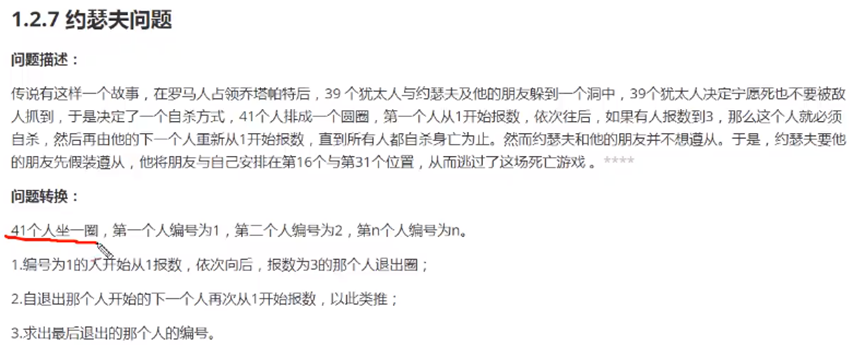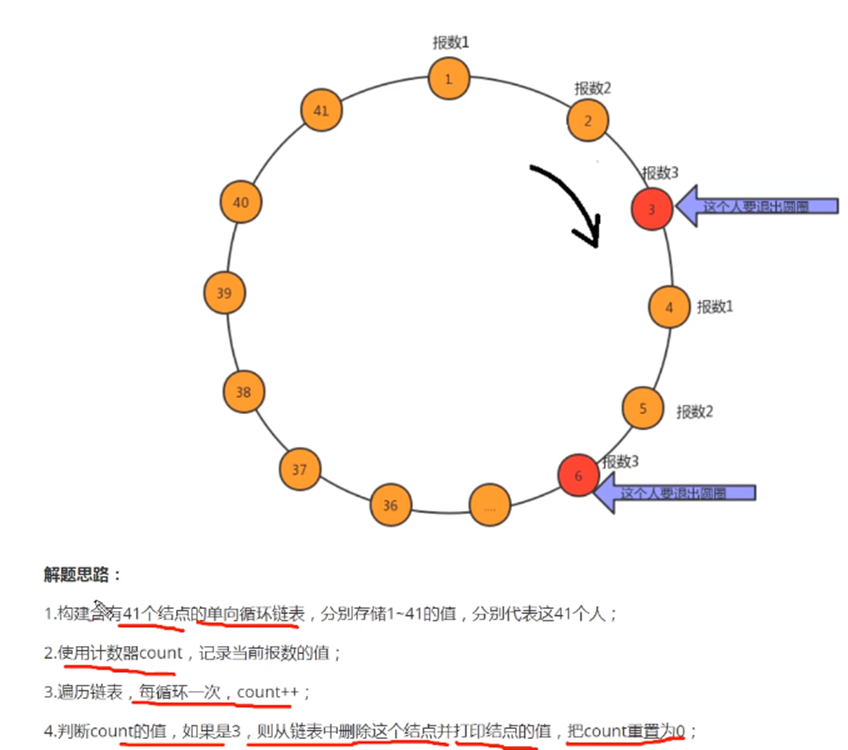﻿• 价格透明
• 信息保密
• 进度掌控
• 售后无忧# 循环链表解决约瑟夫问题

1.问题引入：2.解题思路：3.编写程序：

``````package linkList;

public class YuSeFuTest {

public static void main(String[] args) {
//解决约瑟夫问题
//1.构建循环链表，包含41个节点，分别存储1~41之间的值
Node<Integer> first=null;  //记录首节点
Node<Integer> pre=null;   //记录前一个节点

//开始生成循环链表
for(int i=1;i<=41;i++){
//第一种情况：如果是第一个节点
if(i==1){
first=new Node<Integer>(i,null);
pre=first;
continue;
}
//第二种情况：如果不是第一个节点
Node<Integer> newNode =new Node<Integer>(i,null);
pre.next=newNode;
pre=newNode;
//第三种情况：如果是最后一个节点，那么需要让最后一个节点的下一个节点指向first，就变成循环链表了
if(i==41){
pre.next=first;
}
}
//2.需要count模拟器进行报数
int count=0;
//3.遍历循环链表
//定义一个节点，记录每次循环拿到的节点,初始化为第一个节点
Node<Integer> n=first;
//定义一个节点，记录当前节点的上一个节点
Node<Integer> before=null;
//循环遍历结束的条件是当某一个节点指向它自己时就代表只剩下一个元素了
while(n!=n.next){
//模拟报数
count++;
//判断当前报数是不是3
if(count==3){
//如果是3则把当前节点删除，打印当前节点，重置count=0，让当前节点n后移
before.next=n.next;
System.out.println(n.item+"号跳崖!");
count=0;
n=n.next;
}
else{
//如果不是3，让before变为当前节点，让当前节点后移
before=n;
n=n.next;
}
}
//打印最后一个元素
System.out.println(n.item+"号活下来了!");
}
//创建一个节点类
private static class Node<T>{
T item;
Node next;
public Node(T item,Node next) {
// TODO Auto-generated constructor stub
this.item=item;
this.next=next;
}
}
}

``````### 低价透明### 金牌服务### 信息保密